## Simulating a sample Embed model

Embed comes with hundreds of sample models that are useful for introducing good design practices, as well as providing insight into the types of system models you are or will be creating. Embed sample models are in the Examples menu.

An interesting Embed model to explore is RoomControl, which simulates an HVAC model of single room cooling with an ON/OFF thermostat. It has hysteresis in the controller and accounts for heat production from people in the room.

To run this model

1.    Start Embed.

2.    Select Examples > Applications > HVAC.

3.    Select RoomControl.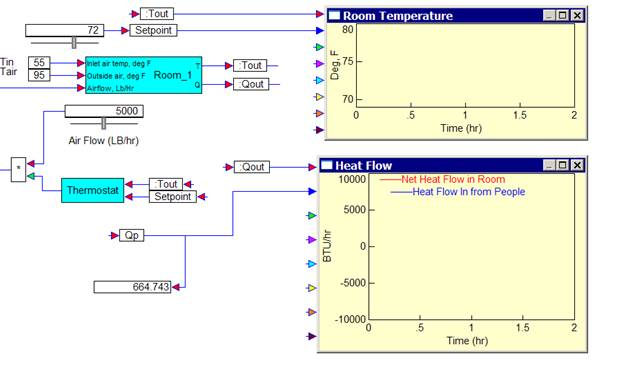4.    To start the simulation, select System > Go, or click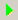.

5.    To stop the simulation, select System > Stop, or click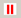.

#### Model properties

Parameters

Qp = rate of heat flow from people

Qin = rate of heat flow carried in by air entering room

Qe = rate of heat flow through room walls

Qnet = sum of all heat flow

Qp0 = heat given off by one person

Troom = room temperature

Tin = temperature of air entering room

Tout = temperature of air leaving room

Tair = temperature of air surrounding room

C = thermal capacitance of air in room

Cr = C + thermal capacitance of furniture and interior walls

R = thermal resistance of walls

w = air flow out of room

S = specific heat of air

A = wall area

P = number of people in room

Equations

Net heat flow (Qnet) is given by:

Qnet = Q p + Qin + Qe

where:

Qp = P * Pp0

Qin = w * S (Tin – Troom)

Qe = (Tair – Troom) * A/R

Substitution yields:

Qnet = P * Qp0 + w *S (Tin – Troom) + (Tair – Troom) * A/R

Room temperature = Qnet/Cr

#### Things to do with RoomControl

This model computes the temperature in a room into which cooled air is flowing. People in the room are used as heat input disturbances.

Setpoint

The setpoint is controlled by a dynamic slider block that specifies the desired temperature in the room.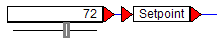You can adjust the temperature as the simulation progresses by sliding the gray rectangular box to the right or left. As you change the setpoint, you can observe how quickly the model responds to the changes. The temperature is initially set to 72 degrees Fahrenheit. The allowable temperature range is 50 to 85 degrees Fahrenheit.

Thermostat

The thermostat is a simple ON/OFF control with hysteresis. It allows fluctuation of 1 degree above the setpoint before turning on the Air Flow to blow cool air into the room. The Air Flow remains on until the temperature drops 1 degree below the setpoint. The temperature at which the thermostat turns ON and OFF around the setpoint is specified by the deadband setting in the thermostat subsystem. It is initially set to 2. You can change the setting to see how the model responds.

Air Flow

The Air Flow is controlled by a dynamic slider block that blows cool air into the room when the thermostat turns ON.

Room

The room is modeled as a simple box with heat flowing in through the walls and heat mass in the room contents and interior walls. There is no heat storage in the room walls and the room air is completely mixed.

The following assumptions are made:

A typical house (1500 sq ft) requires 3 tons of cooling

1 ton of cooling = 12000 BTU/hr

Density of air = 0.076 Lb/f3

400 f3/min = 1 ton of cooling

For 1 ton of cooling, 60*0.076*400 = 1824 Lb/Hr is required

Specific heat of air = 0.24 BTU/(Lb - deg F)

All units in Lb/hr, deg F

Q = heat flow in units of BTU/hr = delta-T*S*w

For cooling

Tin = 55 deg F

Tair = 85 deg F or higher

Troom = Tout = 68 to 75 deg F

People

The number of people entering and exiting the room is a subsystem within the Room subsystem. The number of people is generated by integrating a Gaussian random number function.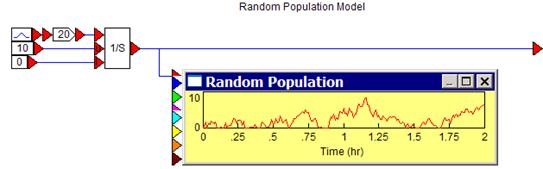Running the simulation

As you run the simulation, you can immediately see the how the temperature fluctuates as people enter and exit the room, as well as when and for how long cool air is blown into the room. By varying the setpoint and air flow, you can see how they affect the time it takes to cool down the room.